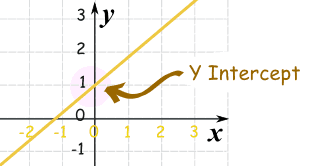# Y – intercept

Standard

## Y Intercept

Where a straight line crosses the Y axis of a graph.

### Example:In the above diagram the line crosses the Y axis at 1.

So the Y intercept is equal to 1.